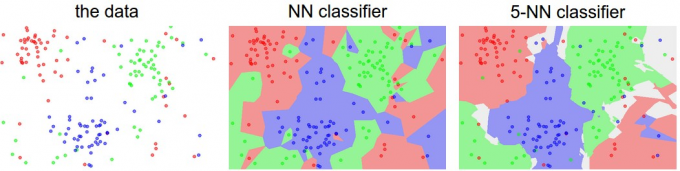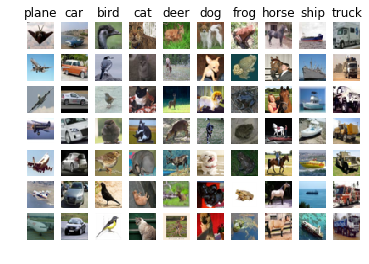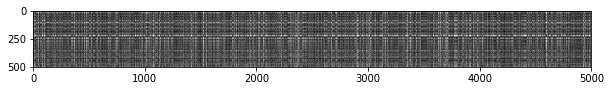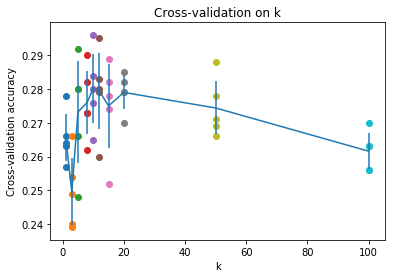$K$ 近邻算法（k-Nearest Neighbor）是CS231n课程介绍的第一个算法，此算法和神经网络没有任何关系，实际中也极少使用，但学习使用KNN算法可以获得对图像分类方法的基本认知。

## 先决条件

### Jupyter Notebook先决条件

Anaconda Prompt Powershell中输入conda activate cs231，接着cdassignment1目录下，输入jupyter notebook开启Ipython笔记本，打开knn.ipynb即可开始本次作业。

### Numpy先决条件

Numpy是CS231n课程中所需的科学计算库，其优秀的矩阵运算性能对图像处理有巨大帮助，在此介绍KNN算法中需使用的Numpy函数。

• 基于元素的运算

Numpy的所有计算都是基于元素的。设AB是两个矩阵，则A + B表示两个矩阵的对应位相乘，A × B同理；而若要作矩阵乘法，让B右乘A，则可以写成A.dot(B)A @ B

• Numpy数组切片

• Numpy幂运算

• np.sum()

• np.sqrt()

• np.argsort()

• np.argmax()

• np.bincount()

## KNN算法

### 思路

KNN算法遵循以下步骤：

• 取CIFAR-10数据集中的一张图片 $（32\times32\times3）$ ，将其拉伸为一个3072维的向量，训练集中的每个向量都可以视作 $3072$ 维欧氏空间中的一个点。

• 对测试集中的每一张图片作相同的操作，计算其与训练集中每一张图的欧式距离。

• 对测试集中的任意一张图片，考察其在训练集中的前 $K$ 近的点（用 $L2$ 距离计算），分类数最大的分类即预测为此图像的所属分类。$d_2(I_1, I_2) = \sqrt{\displaystyle\sum_{p}(I_1^p - I_2^p)^2}$

### 实现

#### 初始化

In

#### 加载数据

In

Out

#### 预处理数据In

Out

#### 两重循环计算 $L2$ 距离

In

Out

In

OutIn

Out

In

Out

#### 一重循环计算 $L2$ 距离

In

Out

#### 纯向量化计算 $L2$ 距离

In

Out

#### 比对三种实现方式的速度

In

Out

### 交叉验证与测试

In

Out

In

OutIn

Out